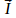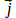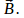## Maxwell's Equations

Maxwell's equations form the basis of electromagnetic theory, allowing the properties of light to be derived. Maxwell's eqiuations are the following:

Gauss's (Coulomb's Law for the Electric Field)

Coulomb's Law gives the force between two point chargesanda distance r apart:This may be extended to find the force between a point charge and a distribution of charges:Gauss's Law for the Magnetic Field

A currentor a current densitycreates a magnetic field#### Add commentRefresh